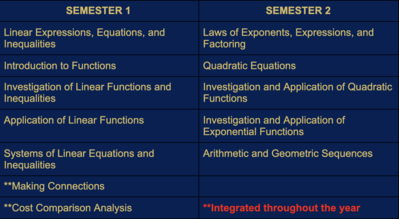# MS Math

•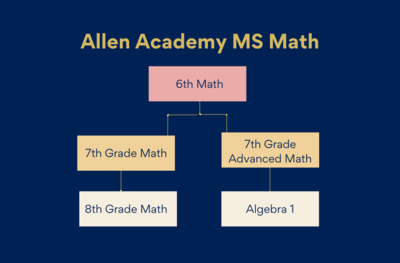The basic operations of addition, subtraction, multiplication, and division of whole numbers, fractions, and decimals will be studied with an emphasis on using these skills to solve problems in the real world. Students will utilize different problem solving techniques that include collecting, organizing, and interpreting data, making charts and tables, graphing, estimation, judging the reasonableness of an answer, use of technology when appropriate, finding a pattern, and writing an equality or inequality to represent the problem. Geometrical concepts and their use in the real world will be addressed.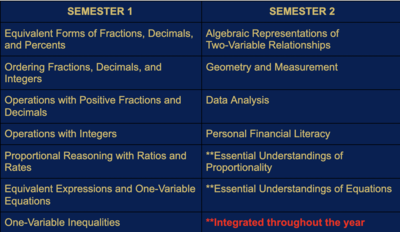The primary focal points at 7th grade are number and operations; proportionality; expressions, equations, and relationships; and measurement and data. Students use concepts, algorithms, and properties of rational numbers to explore mathematical relationships and to describe increasingly complex situations.Advanced Math is a combination of 7th grade and 8th grade TEKS.  Students will engage in rigorous study of algebraic and graphical representations of problems; function notation and language; linear, quadratic, and exponential relationships; data regression; and the real number system including both rational and irrational numbers.

**This class is based on teacher recommendation and 6th grade data. Instruction will move at a rapid pace in order to ensure students receive foundational knowledge and understand concepts necessary for future math classes. Students will be allowed to opt out of Advanced Math before the end of the first quarter, with parent consent.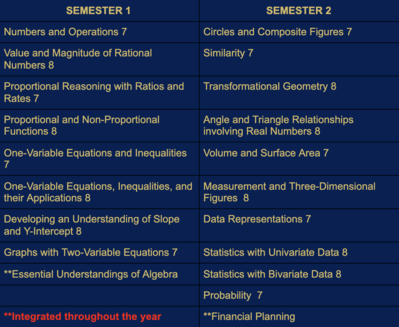The primary focal points at 8th grade are proportionality; expressions, equations, relationships, and foundations of functions; and measurement and data.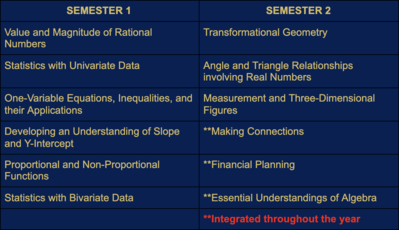Algebra 1

In Algebra I the fundamental purpose of this course is to formalize and extend the mathematics that students learned in the middle grades. Instruction will focus on five critical areas: (1) analyze and explain the process of solving equations and inequalities: (2) learn function notation and develop the concepts of domain and range; (3) use regression techniques; (4) create quadratic and exponential expressions; and (5) select from among these functions to model phenomena.

**This class is based on teacher recommendation and 7th grade data.

**Students will receive high school credit for this course, however, it will not be calculated toward their GPA.